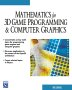# Maths - Matrix algebra - 3D arithmatic

The following calculator allows you to calculate 3x3 matrix arithmetic. Enter the values into the matrix into the top two matries and then press "+ - or * " to display the result in the bottom matrix:

=

To add matrices just add the corresponding elements, so for 3x3 matrices and then,

 a00 + b00 a01 + b01 a02 + b02 a10 + b10 a11 + b11 a12 + b12 a20 + b20 a21 + b21 a22 + b22
=
 a00 a01 a02 a10 a11 a12 a20 a21 a22
+
 b00 b01 b02 b10 b11 b12 b20 b21 b22

### Matrix Subtraction

To subtract matrices just subtract the corresponding elements, so for 3x3 matrices and then,

 a00 - b00 a01 - b01 a02 - b02 a10 - b10 a11 - b11 a12 - b12 a20 - b20 a21 - b21 a22 - b22
=
 a00 a01 a02 a10 a11 a12 a20 a21 a22
-
 b00 b01 b02 b10 b11 b12 b20 b21 b22

### Matrix Multiplication

The multiplication of two 3x3 matrices is:

 a00*b00 + a01*b10 + a02*b20 a00*b01 + a01*b11 + a02*b21 a00*b02 + a01*b12 + a02*b22 a10*b00+ a11*b10 + a12*b20 a10*b01 + a11*b11 + a12*b21 a10*b02 + a11*b12 + a12*b22 a20*b00 + a21*b10 + a22*b20 a20*b01 + a21*b11 + a22*b21 a20*b02 + a21*b12 + a22*b22
=
 a00 a01 a02 a10 a11 a12 a20 a21 a22
 b00 b01 b02 b10 b11 b12 b20 b21 b22

This is worked out as follows: for each entry in the matrix we take the row from the first operand and the column from the second operand:

 a00 a01 a02 a10 a11 a12 a20 a21 a22
 b00 b01 b02 b10 b11 b12 b20 b21 b22
=
 a00 a01 a02
 b00 b10 b20
 a00 a01 a02
 b01 b11 b21
 a00 a01 a02
 b02 b12 b22
 a10 a11 a12
 b00 b10 b20
 a10 a11 a12
 b01 b11 b21
 a10 a11 a12
 b02 b12 b22
 a20 a21 a22
 b00 b10 b20
 a20 a21 a22
 b01 b11 b21
 a20 a21 a22
 b02 b12 b22

This single row times a single column is equivalent to the dot product.

 metadata block see also: Correspondence about this page Book Shop - Further reading. Where I can, I have put links to Amazon for books that are relevant to the subject, click on the appropriate country flag to get more details of the book or to buy it from them.Mathematics for 3D game Programming - Includes introduction to Vectors, Matrices, Transforms and Trigonometry. (But no euler angles or quaternions). Also includes ray tracing and some linear & rotational physics also collision detection (but not collision response). Specific to this page here:

This site may have errors. Don't use for critical systems.